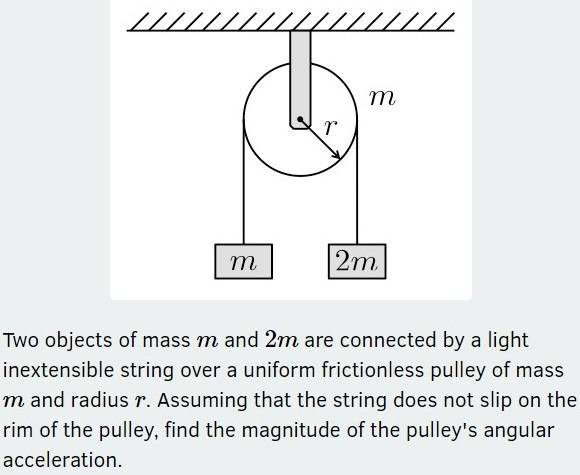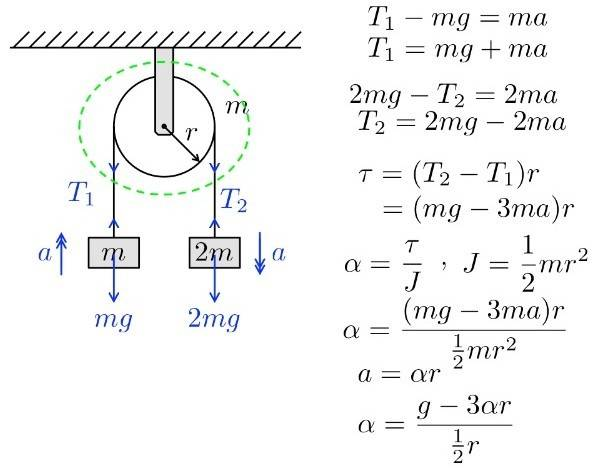# Moment of Inertia with pulley and two masses on a string (iWTSE.org)

TomK
Homework Statement:
Relevant Equations:
torque = inertia x angular acceleration

angular acceleration = linear acceleration / radius (of curved trajectory)

torque = force x perpendicular distance (between a chosen point and line of force direction)Note: the working (taken from iWTSE website) refers to inertia as the symbol ‘J’ (in-case there was any confusion).

I found equations of motion for mass m and 2m which were ‘T1 = ma + mg’ and ‘T2 = 2mg – 2ma’, respectively. I know they are connected particles with the same acceleration ‘a’.

In this problem, do we assume the circular pulley is a thin ring with inertia = (1/2)mr2? I looked up the inertia of different shapes, and it seems like a solid disk would, instead, be (1/4)mr2. I’m assuming the problem wants me to think of the pulley as a hollow ring.

How can we prove that a hollow ring has inertia = (1/2)mr2?

Why does this video (at 5:35) say that a wheel had moment of inertia = mr2? If this is correct, what shape is the wheel (in the video) meant to represent (is it a solid disk, a hollow ring, or something else)?

I want to clarify why the working shows a net force of 'T2 – T1', in the ‘torque = Fr’ equation. Is it because T2 is pointing down and the pulley is going to rotate in a clockwise direction?

•Delta2

Homework Helper
Gold Member
The moment of inertia depends not only on the shape and distribution of mass across the shape , but also on the choice of axis of rotation.

For a thin disk, of uniform mass density (mass is equally distributed across the shape of the disk) and for an axis of rotation perpendicular to the plane of the disk and passing through its center the moment of inertia is ##\frac{1}{2}mr^2##. This is the case of the rotating pulley.

If we take the axis of rotation to be instead along any radius of the disk, then for the same shape and mass distribution the moment of inertia is ##\frac{1}{4}mr^2##

Take a look at the following link for a list of moment of inertia of the most simple shapes. For some shapes it lists 2 or more results, depending on the choice of axis of rotation.
https://en.wikipedia.org/wiki/List_of_moments_of_inertia

•Lnewqban and TSny
TomK
The moment of inertia depends not only on the shape and distribution of mass across the shape , but also on the choice of axis of rotation.

For a thin disk, of uniform mass density (mass is equally distributed across the shape of the disk) and for an axis of rotation perpendicular to the plane of the disk and passing through its center the moment of inertia is ##\frac{1}{2}mr^2##. This is the case of the rotating pulley.

If we take the axis of rotation to be instead along any radius of the disk, then for the same shape and mass distribution the moment of inertia is ##\frac{1}{4}mr^2##

Take a look at the following link for a list of moment of inertia of the most simple shapes. For some shapes it lists 2 or more results, depending on the choice of axis of rotation.
https://en.wikipedia.org/wiki/List_of_moments_of_inertia

Is there a way to derive a shape's moment of inertia formula (does it go into more-complex mathematics that I wouldn't understand yet)? Is the video I linked to incorrect at timestamp 5:35?

As for the resultant force, is it 'T2 - T1' because T2 aids in the pulley rotating clockwise whereas T1 acts anticlockwise? Is that the correct reason?

Homework Helper
Gold Member
That moment of inertia belongs to a solid disc rotating about an axis parallel perpendicular to its plane.

http://hyperphysics.phy-astr.gsu.edu/hbase/mi.html#mi

Imagine three blocks on a horizontal surface connected by two strings instead.
The linear inertia of that middle block would affect the magnitudes of T1and T2, as well as the acceleration (a) of the whole system in the direction toward which the resultant external force is acting (down from the mass located on the right side of the pulley in the given configuration).

Last edited:
TomK
That moment of inertia belongs to a solid disc rotating about an axis parallel to its plane.

http://hyperphysics.phy-astr.gsu.edu/hbase/mi.html#mi

Imagine three blocks on a horizontal surface connected by two strings instead.
The linear inertia of that middle block would affect the magnitudes of T1and T2, as well as the acceleration (a) of the whole system in the direction toward which the resultant external force is acting (down from the mass located on the right side of the pulley in the given configuration).

In the original problem, why do we know that the pulley is rotating about its diameter, as opposed to its rotational axis?

Was the reason I gave right for explaining the resultant force?

Homework Helper
Gold Member
In the original problem, why do we know that the pulley is rotating about its diameter, as opposed to its rotational axis?

Was the reason I gave right for explaining the resultant force?
Sorry, I meant to write that ##I=0.5mr^2## belongs to a solid disc rotating about its symmetry axis.

The resultant force is a subtraction of vectors, since they have opposite directions of action.

•Delta2
TomK
Thank you for answering all my questions. I understand the question now.

•Lnewqban
Homework Helper
Gold Member
You are welcome, Tom.Homework Helper
Gold Member
Is there a way to derive a shape's moment of inertia formula (does it go into more-complex mathematics that I wouldn't understand yet)? Is the video I linked to incorrect at timestamp 5:35?
The video is correct but it models the wheel as a hollow ring and also choose the axis of rotation passing through the center of the ring and perpendicular to the plane of the ring.
Also the video is correct when it says that the moment of inertia is this integral $$\int r^2 dm$$ but this integral the way it is written is a bit abbreviated. I think that the most correct and detailed way to write this integral is $$\iiint_Q r^2 \rho(x,y,z) dxdydz$$
Things to note about the above integral:
• It is a triple (volume) integral, i gave it in cartesian coordinates but you can convert it to spherical or cylindrical coordinates. It is a triple integral cause all bodies have three dimensions at least in our universe :) In some cases we simplify it to a double or single integral (like for example when we want to calculate the MoI of a thin disk, because the disk is thin it has negligible thickness in the z-dimension so we can omit the z-coordinate from the integral and do a double integration).
• Q is the domain of integration and it is defined by the shape of the body. This domain Q usually help us decide which choice of coordinate system (cartesian, cylindrical or spherical or other) we will choose to perform the integration
• ##r## is the perpendicular distance of the mass element ##dm=\rho(x,y,z) dxdydz## at the point (x,y,z), from the axis of rotation, and it is not the "r" of the spherical or cylindrical coordinate system. The choice of axis of rotation affect which is the exact expression (involving the coordinates x,y,z in the case of cartesian system) that equals ##r## so it is ##r=f(x,y,z)## for a proper function f that gives us the aforementioned distance
• ##\rho (x,y,z) ## is the mass density of the body at the point (x,y,z)
As for the resultant force, is it 'T2 - T1' because T2 aids in the pulley rotating clockwise whereas T1 acts anticlockwise? Is that the correct reason?
Yes!

•Lnewqban